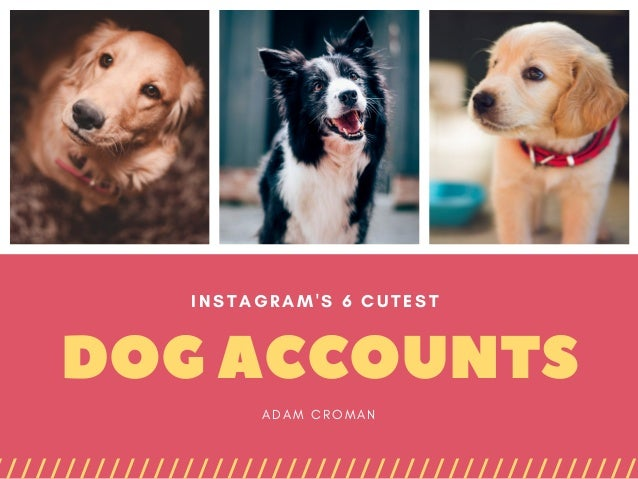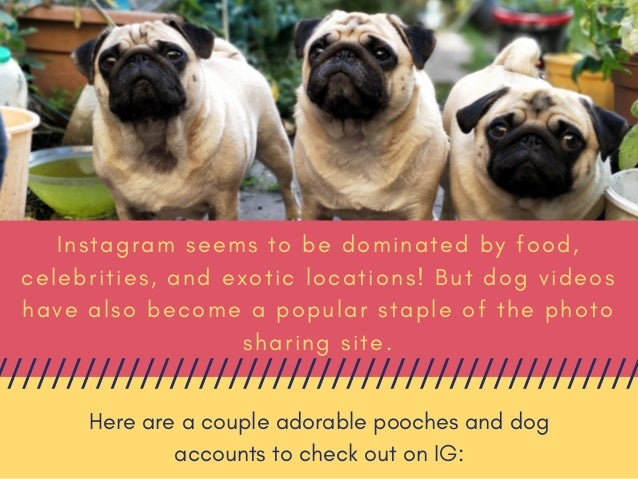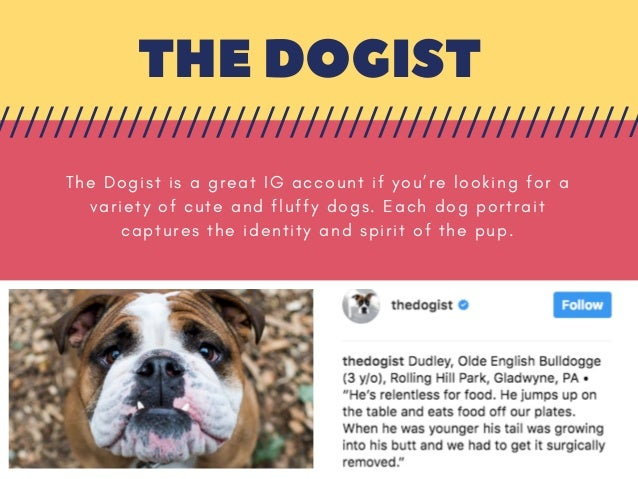Successfully reported this slideshow.Upcoming SlideShare
×

ofUpcoming SlideShare
Next

0 Likes

Share

# Instagram's 6 Cutest Dog Accounts

Instagram seems to be dominated by food, celebrities, and exotic locations! But dog videos have also become a popular staple of the photo sharing site.

See all

### Related Audiobooks

#### Free with a 30 day trial from Scribd

See all
• Be the first to like this

### Instagram's 6 Cutest Dog Accounts

1. 1. DOGACCOUNTS INSTAGRAM'S 6 CUTEST  A D A M C R O M A N
2. 2. I n s t a g r a m s e e m s t o b e d o m i n a t e d b y f o o d , c e l e b r i t i e s , a n d e x o t i c l o c a t i o n s ! B u t d o g v i d e o s h a v e a l s o b e c o m e a p o p u l a r s t a p l e o f t h e p h o t o s h a r i n g s i t e . Here are a couple adorable pooches and dog accounts to check out on IG:
3. 3. THEDOGIST T h e D o g i s t i s a g r e a t I G a c c o u n t i f y o u ’ r e l o o k i n g f o r a v a r i e t y o f c u t e a n d f l u f f y d o g s . E a c h d o g p o r t r a i t c a p t u r e s t h e i d e n t i t y a n d s p i r i t o f t h e p u p .
4. 4. LOKITHEWOLFDOG Elegant and whimsical, Loki is a beautiful husky/Arctic wolf/Malamute hybrid. Followers often find photos of Loki sledding, climbing, camping, or relaxing on the side of a mountain.
5. 5. SAMSONTHEDOOD A B r o o k l y n , N e w Y o r k n a t i v e , t h e I n s t a g r a m s t a r n a m e d S a m s o n t h e D o o d i s a s c u t e a n d c u d d l y a s c a n b e f o r a l o v a b l e p o o c h ( w h i c h i s a l o t ! ) . S a m s o n i s a g o l d e n r e t r i e v e r a n d p o o d l e m i x ( c a l l e d a g o l d e n d o o d l e ) w h o w e l o v e w a t c h i n g g r o w u p !
6. 6. MARNIETHEDOG A s o n e o f t h e m o s t p o p u l a r d o g s o n I n s t a g r a m , t h e 1 5 - y e a r - o l d S h i h T z u i s b e l o v e d b y m a n y f o r h e r c u t e n e s s , b u t a l s o h e r r a g s - t o - r i c h e s s t o r y .
7. 7. DOUGTHEPUG D o u g t h e P u g i s o n e o f t h e m o s t w e l l - k n o w n I n s t a g r a m d o g s o u t t h e r e t o d a y . A n d h e ’ s a l s o o n e o f t h e c o o l e s t .
8. 8. PUPPYSTAGRAM This endearing Instagram account offers us great posts of puppies galore! Looking for a post of a chubby, furry pup whose face you just want to smother with love?

Total views

1,368

On Slideshare

0

From embeds

0

Number of embeds

60

0

Shares

0